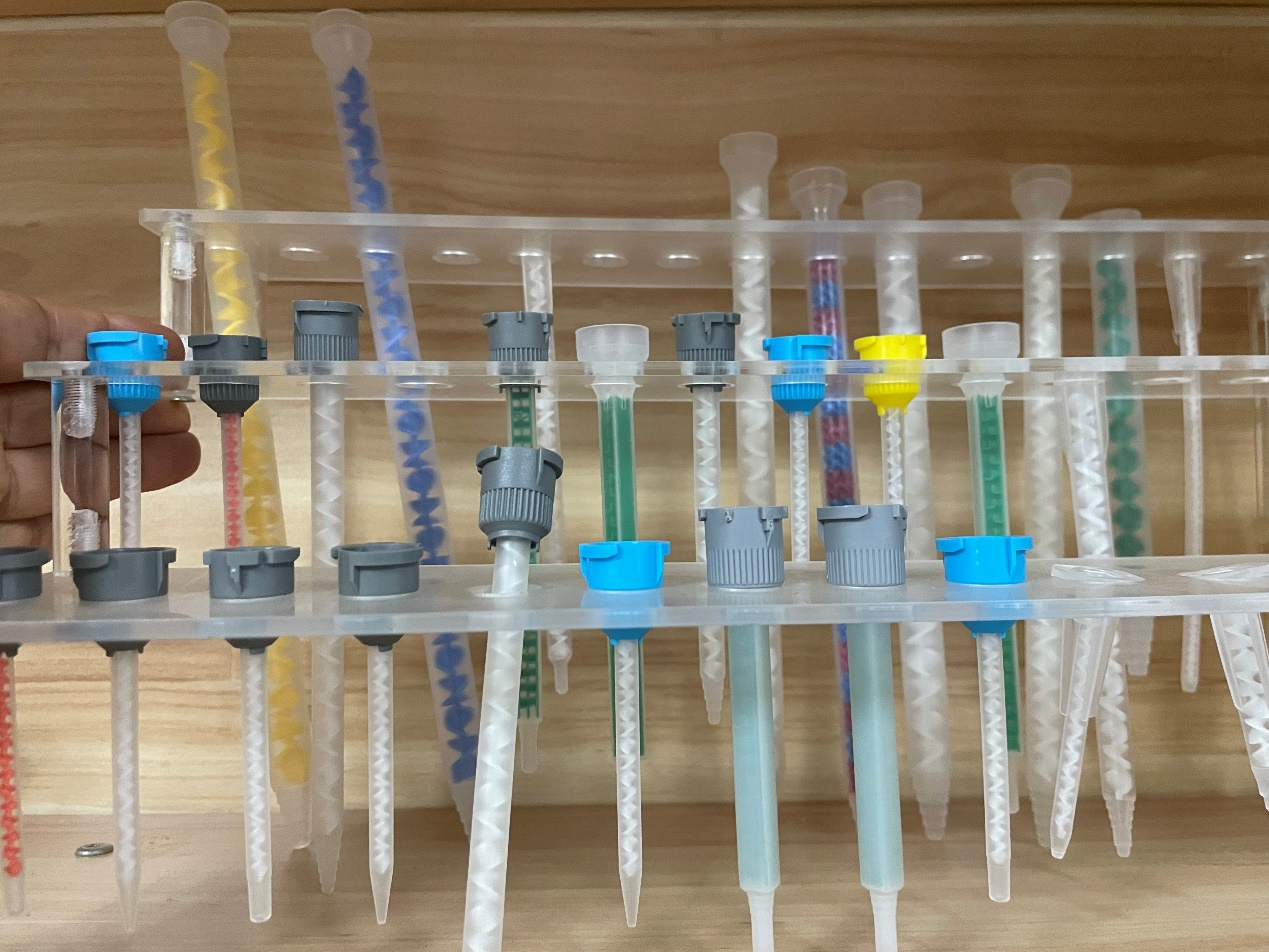English» » How to determine a mixer size？

## Main Products

Send Us A Message

# How to determine a mixer size？

Views: 2     Author: Site Editor     Publish Time: 2023-04-11      Origin: SiteCalculate the flow rate: Determine the flow rate of the fluid being mixed in units of volume per time, such as gallons per minute or liters per second. This value will be used to calculate the diameter of the mixer.

Determine the Reynolds number: The Reynolds number is a dimensionless value that represents the ratio of inertial forces to viscous forces. It is calculated as Re = (ρVD)/μ, where ρ is the density of the fluid, V is the velocity of the fluid, D is the diameter of the mixer, and μ is the viscosity of the fluid. The Reynolds number is used to determine the required mixer length and configuration.

Determine the mixer diameter: The diameter of the mixer is calculated using the following equation: D = (Q/πV), where Q is the flow rate and V is the velocity of the fluid.

Calculate the required mixer length: The required length of the mixer depends on the Reynolds number and the desired mixing quality. The length of the mixer can be calculated using empirical correlations or simulation models. Typically, longer mixers provide better mixing quality than shorter mixers.

Select the mixer design: The mixer design is selected based on the fluid properties and the desired mixing quality. Different designs offer different mixing characteristics, such as laminar flow, turbulent flow, or shear. Some common designs include helical, Kenics, and static mixer plates.

Verify the mixer size: Once the mixer diameter and length have been calculated, it is important to verify that the selected mixer size is appropriate for the application. This can be done by testing the mixer in the actual application or by using simulation models.